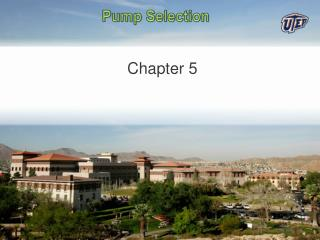DownloadDownload PresentationPump Selection

# Pump Selection

Télécharger la présentation## Pump Selection

- - - - - - - - - - - - - - - - - - - - - - - - - - - E N D - - - - - - - - - - - - - - - - - - - - - - - - - - -
##### Presentation Transcript

1. Pump Selection Chapter 5

2. Think of a sprinkler head, except you turn the sprinkler to move the water rather then the other way around Centrifugal Pump Concept

3. Principle of angular momentum conservation • Momentum=mass*velocity • Angular momentum=radius*mass*velocity • Torque=time rate of change of angular momentum

4. PUMPING ENERGY • Power=Qhpump • = Q (P2-P1) • The mechanical horsepower, also known as imperial horsepower, of exactly 550 foot-pounds per second is approximately equivalent to 745.7 watts.

5. Types of Pumps • Propeller, used for low head (<12 m), high capacity (>20L/s)

6. Multistage propeller • Used in deep wellswith high head and lowflow rate

7. Jet Pumps • Take energy from high pressure fluid • No moving parts • Compact and light but not efficient • Q1 is high pressure fluid • Q2 is fluid to be pumped

8. Positive Displacement Pumps

9. Positive Displacement Pump • Very high head, low flow

10. How to size a pump • To find operating point: • obtain pump curve from manufacturer, pumps pump less water at higher heads • calculate the system curve, the system curve is an equation that tells how much water flows through a pipe system as a function of the head (energy per unit weight) added by the pump. • Energy efficiency is maximized when the operation point (intersection of pump and system curves) is at the point of maximum pump efficiency

11. Cavitation is Evil • Cavitation can occur whenever the absolute pressure locally gets below the vapor pressure of the water • Frequent issue when pump is higher than water reservoir but can occur with any pump  • Common site of cavitation is near tips of impellor vanes where velocity is high

12. Pump Selection

13. Pump Tips • http://www.pumpfundamentals.com/centrifugal-pump-tips.htm

14. Pump Fundamentals • http://www.pumpfundamentals.com/tutorial2.htm#pump-pressure • http://www.pumpworld.com/Capacity.htm

15. How to size a pump • To find operating point: • obtain pump curve from manufacturer, pumps pump less water at higher heads • calculate the system curve, the system curve is an equation that tells how much water flows through a pipe system as a function of the head (energy per unit weight) added by the pump. • Energy efficiency is maximized when the operation point (intersection of pump and system curves) is at the point of maximum pump efficiency

16. Brake horsepower (bhp) is the measure of an engine's horsepower before the loss in power caused by the gearbox, alternator, differential, water pump, and other auxiliary components Explain Pump Curve System curve

17. How to size a pump • To find operating point: • obtain pump curve from manufacturer, pumps pump less water at higher heads • calculate the system curve, the system curve is an equation that tells how much water flows through a pipe system as a function of the head (energy per unit weight) added by the pump. • Energy efficiency is maximized when the operation point (intersection of pump and system curves) is at the point of maximum pump efficiency

18. Example System • Show using Bernoulli Equation (good quiz!) • Hpump=z2-z1+hloss • This is the system curve for our simple system shown below

19. Solution where curves intersect

20. Pumps in Parallel – Add Discharge